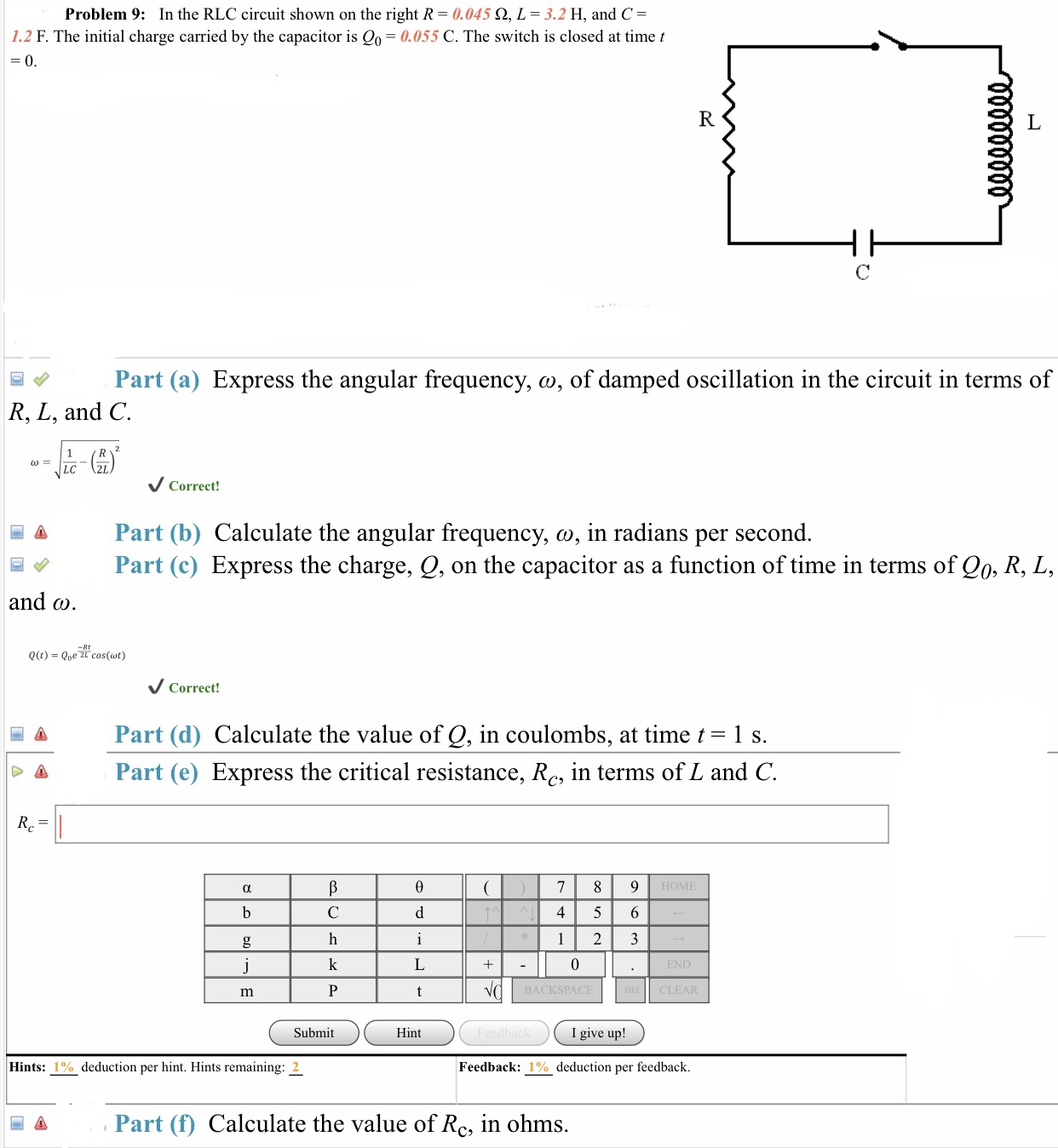# Problem 9: In the RLC circuit shown on the right R-0.045 2, L 3.2 H, and C-1.2 F. The initial charge carried by the capacitor is o 0.055 C. The switch is closed at time tPart (a) Express the angular frequency, ω, of damped oscillation in the circuit in terms ofR, L, and CCorrect!A Part (b) Calculate the angular frequency, o, in radians per second.Part (c) Express the charge, Q, on the capacitor as a function of time in terms of Qo, R, L,and ωCorrect!A Part (d) Calculate the value of Q, in coulombs, at time t-1 s.Part (e) Express the critical resistance, Rc, in terms of L and CHOM4 5 6BACKSPACECLEARSubmitHintI give up!Hints: 1 %) deduction per hint. Hints remaining: 2Feedback: 1% deduction per feedback.Part (f) Calculate the value of Rc, in ohms.

Question

B,D,Ehelp_outlineImage TranscriptioncloseProblem 9: In the RLC circuit shown on the right R-0.045 2, L 3.2 H, and C- 1.2 F. The initial charge carried by the capacitor is o 0.055 C. The switch is closed at time t Part (a) Express the angular frequency, ω, of damped oscillation in the circuit in terms of R, L, and C Correct! A Part (b) Calculate the angular frequency, o, in radians per second. Part (c) Express the charge, Q, on the capacitor as a function of time in terms of Qo, R, L, and ω Correct! A Part (d) Calculate the value of Q, in coulombs, at time t-1 s. Part (e) Express the critical resistance, Rc, in terms of L and C HOM 4 5 6 BACKSPACE CLEAR Submit Hint I give up! Hints: 1 %) deduction per hint. Hints remaining: 2 Feedback: 1% deduction per feedback. Part (f) Calculate the value of Rc, in ohms. fullscreen
check_circle

Step 1

Given: R = 0.045ohm, L = 3.2 H, C = 1.2 F and initial charge of the capacitor Q0 = 0.055 C.

Therefore, the angular frequency, ω, of damped oscillation in the circuit in terms of R, L, and C will be:

Step 2

(b) The  angular frequency will be:

Step 3

(c) The charge, Q, on the capacitor as a function of t...

### Want to see the full answer?

See Solution

#### Want to see this answer and more?

Solutions are written by subject experts who are available 24/7. Questions are typically answered within 1 hour.*

See Solution
*Response times may vary by subject and question.
Tagged in

### Current Electricity